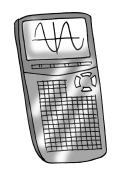### Home > INT1 > Chapter 10 > Lesson 10.1.3 > Problem10-53

10-53.

The Dallas University Health Center is running a clinical trial comparing two medicines to treat fibromyalgia. They need to compare the ages of the two groups receiving the medicines. The ages follow:Group 7A: $72$, $71$, $59$, $62$, $48$, $70$, $57$, $48$, $68$, $68$, $57$, $49$, $53$, $72$, $60$
checksum $914$

Group 7B: $64$, $29$, $75$, $55$, $85$, $51$, $74$, $34$, $80$, $28$, $49$, $79$, $68$, $45$, $94$, $32$
checksum $942$

1. Use your calculator to make a sketch of the combination histogram and boxplot for each treatment group. Sketch the graphs in your notebook. Compare and contrast the distributions of ages between the two groups using the center, shape, spread, and outliers of the two groups.

Use either the mean or the median to describe the center.
Use the IQR to describe the spread.
Are there any apparent outliers?

2. When reporting the typical age in each group, which is a more appropriate measure of center, the mean or the median? Explain.

Recall that outliers affect the mean more than the median.
Are there any outliers?

Enter the data in the eTool below to solve the problem.
Click the link at right for the full version of the eTool: Int1 10-53 HW eTool.